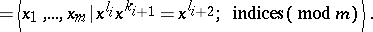# Fibonacci group

The Fibonacci group $F(2,m)$ has the presentation (cf. also Finitely-presented group; Presentation):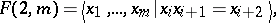where indices are taken modulo.

Fibonacci groups were introduced by J.H. Conway [a2] and are related to the Fibonacci numbers with inductive definition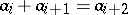(with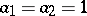as initial ones).

Several combinatorial studies (see [a1] for references) answered some questions on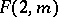, including their non-triviality and finiteness:is finite only for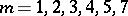. H. Helling, A.C. Kim and J. Mennicke [a3] provided a geometrization of, by showing that the groups,, are the fundamental groups of certain closed orientable three-manifolds (so-called Fibonacci manifolds, denoted by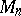). See also Fibonacci manifold. In fact, for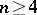,, whereis a closed hyperbolic three-manifold;, whereis the Euclidean Hantzche–Wendt manifold;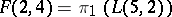, with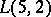a lens space.

This and properties of the fundamental groups of these three-manifolds imply that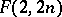are Noetherian groups, i.e. every finitely-generated subgroup ofis finitely presented (cf. also Noetherian group). Sinceis an affine Riemannian manifold,is a torsion-free finite extension of. Due to hyperbolicity for(cf. also Hyperbolic group), theare torsion-free, their Abelian subgroups are cyclic (cf. also Cyclic group), there are explicit imbeddings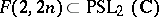, and the word and conjugacy problems are solvable for them (cf. also Group calculus; Identity problem). Also, the groups,, are arithmetic if and only if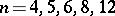; see [a3], [a4] and Arithmetic group.

There are several generalizations of Fibonacci groups, related to generalizations of Fibonacci numbers. D.L. Johnson [a5] has introduced the generalized Fibonacci groups (see [a9] for a survey)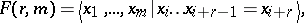where indices are taken modulo. Another generalization of Fibonacci groups is due to C. Maclachlan [a7] (see [a8] for their geometrization):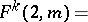Fractional Fibonacci groups were introduced by A.C. Kim and A. Vesnin in [a6] (which contains their geometrization as well):